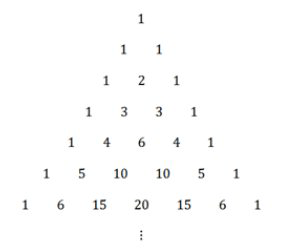# 2.3: 2.3 Polynomial Expansion and Pascal's Triangle

•• Contributed by CK12
• CK12

The expression $$(2 x+3)^{5}$$ would take a while to multiply out. Is there a pattern you can use?

### Expansion with Pascal's Triangle

#### Expanding a Binomial

Pascal was a French mathematician in the $$17^{t h}$$ century, but the triangle now named Pascal's Triangle was studied long before Pascal used it. The pattern was used around the $$10^{t h}$$ century in Persia, India and China as well as many other places.The primary purpose for using this triangle is to introduce how to expand binomials.

$$(x+y)^{0}=1$$
$$(x+y)^{1}=x+y$$
$$(x+y)^{2}=x^{2}+2 y+y^{2}$$
$$(x+y)^{3}=x^{3}+3 x^{2} y+3 x y^{2}+y^{3}$$

Notice that the coefficients for the $$x$$ and $$y$$ terms on the right hand side line up exactly with the numbers from Pascal's triangle. This means that given $$(x+y)^{n}$$ for any power $$n$$ you can write out the expansion using the coefficients from the triangle. Note that to write the coefficients for any power $$n$$, you need to look at row $$n+1$$ to find the coefficients.

If you were asked to expand $$(3 x-2)^{4}$$ using Pascal's Triangle, you would look at the 5 th row to find the coefficients. The coefficients will be 1,4,6,4,$$1 ;$$ however, since there are already coefficients with the $$x$$ and the constant term you must be particularly careful.

$$1 \cdot(3 x)^{4}+4 \cdot(3 x)^{3} \cdot(-2)+6 \cdot(3 x)^{2} \cdot(-2)^{2}+4 \cdot(3 x) \cdot(-2)^{3}+1 \cdot(-2)^{4}$$

Then it is only a matter of multiplying out and keeping track of negative signs.

$$81 x^{4}-216 x^{3}+216 x^{2}-96 x+16$$

When you study how to count with combinations then you will be able to calculate the value of any coefficient without writing out the whole triangle.

#### Patterns and Pascal's Triangle

There are many patterns in the triangle.  Here are just a few.1. Notice the way each number is created by summing the two numbers above on the left and right hand side.
2. As you go further down the triangle the values in a row approach a bell curve. This is closely related to the normal distribution in statistics.
3. For any row that has a second term that is prime, all the numbers besides 1 in that row are divisible by that prime number.

Real Life Use: In the game Plinko where an object is dropped through a triangular array of pegs, the probability (which corresponds proportionally to the values in the triangle) of landing towards the center is greater than landing towards the edge. This is because every number in the triangle indicates the number of ways a falling object can get to that space through the preceding numbers.### Examples

Example 1

Earlier, you were asked to multiply out $$(2 x+3)^{5}$$. Pascal's triangle allows you to identify that the coefficients of $$(2x+3)^{5}$$ will be $$1,5,10,10,5,1 .$$ By carefully substituting, the expansion will be:

$$1 \cdot(2 x)^{5}+5 \cdot(2 x)^{4} \cdot 3+10 \cdot(2 x)^{3} \cdot 3^{2}+10 \cdot\left(2 x^{2}\right) \cdot 3^{3}+5(2 x)^{1} \cdot 3^{4}+3^{5}$$

Simplifying is a matter of arithmetic, but most of the work is done thanks to the patterns of Pascal’s Triangle.

Example 2

Factor the following polynomial by recognizing the coefficients.

$$x^{4}+4 x^{3}+6 x^{2}+4 x+1$$

The coefficients are 1, 4, 6, 4, and 1 and those coefficients are on the 5th row. The first row of Pascal's Triangle shows the coefficients for the 0th power so the 5th row shows the coefficients for the 4th power. Thus, the factored form is:

$$(x+1)^{4}$$

Example 3

Factor the following polynomial by recognizing the coefficients.

$$8 x^{3}-12 x^{2}+6 x-1$$

since the first coefficient is not 1 , you need to take the appropriate root of the first term of the expression to find the first term of the binomial. In this case, the first term of the binomial must be $$2 x$$. Also, the last term must be -1 and the power must be $$3 .$$ Now all that remains is to check.

$$(2 x-1)^{3}=(2 x)^{3}+3(2 x)^{2} \cdot(-1)+3(2 x)^{1}(-1)^{2}+(-1)^{3}=8 x^{3}-12 x^{2}+6 x-1$$

Example 4

Expand the following binomial: $$\left(\frac{1}{2} x-3\right)^{5}$$

You know that the coefficients will be 1, 5, 10, 10, 5, 1.

$$1\left(\frac{1}{2} x\right)^{5}+5\left(\frac{1}{2} x\right)^{4}(-3)+10\left(\frac{1}{2} x\right)^{3}(-3)^{2}+10\left(\frac{1}{2} x\right)^{2}(-3)^{3}+5\left(\frac{1}{2} x\right)(-3)^{4}+1 \cdot(-3)^{5}$$

$$=\frac{x^{5}}{32}-\frac{15 x^{4}}{16}+\frac{90 x^{3}}{8}-\frac{270 x^{2}}{4}+\frac{405 x}{2}-243$$

Remember to simplify fractions.

$$=\frac{x^{5}}{32}-\frac{15 x^{4}}{16}+\frac{45 x^{3}}{4}-\frac{135 x^{2}}{2}+\frac{405 x}{2}-243$$

Example 5

Expand the following trinomial: $$(x+y+z)^{4}$$

Unfortunately, Pascal's triangle does not apply to trinomials. Instead of thinking of a two dimensional triangle, you would ned to calculate a three dimensional pyramid which is called Pascal's Pyramid. The sum of all five terms below is your answer.

$$1 x^{4}+4 x^{3} z+6 x^{2} z^{2}+4 x z^{3}+1 z^{4}$$
$$4 x^{3} y+12 x^{2} y z+12 x y z^{2}+4 y z^{3}$$
$$6 x^{2} y^{2}+12 x y^{2} z+6 y^{2} z^{2}$$
$$4 x y^{3}+4 y^{3} z$$
$$1 y^{4}$$

Notice how many patterns exist in the coefficients of this layer of the pyramid.

Review

Factor the following polynomials by recognizing the coefficients.

1. $$x^{2}+2 x y+y^{2}$$

2. $$x^{3}+3 x^{2}+3 x+1$$

3. $$x^{5}+5 x^{4}+10 x^{3}+10 x^{2}+5 x+1$$

4. $$27 x^{3}-27 x^{2}+9 x-1$$

5. $$x^{3}+12 x^{2}+48 x+64$$

Expand the following binomials using Pascal’s Triangle.

6. $$(2 x-3)^{3}$$

7. $$(3 x+4)^{4}$$

8. $$(x-y)^{7}$$

9. $$(a+b)^{10}$$

10. $$(2 x+5)^{5}$$

11. $$(4 x-1)^{4}$$

12. $$(5 x+2)^{3}$$

13. $$(x+y)^{6}$$

14. $$(3 x+2 y)^{3}$$

15. $$(5 x-2 y)^{4}$$